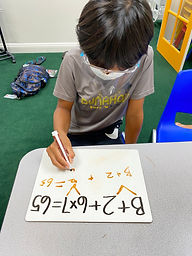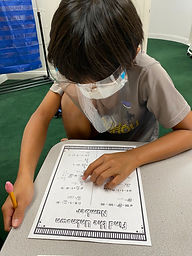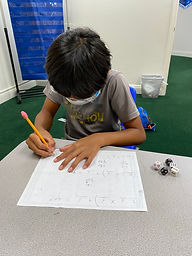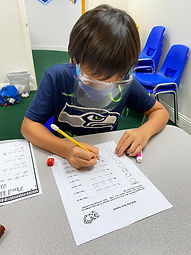## Ms. Amanda

### Target 1​

###### Lesson Type:

Continuation

Algebra

:

Variable

Solve equations that use variables to represent unknown numbers.

###### 1:

Identify the unknown information in problems.

4th

###### Vocabulary:

Unknown

Activities:

- Students solved for unknown numbers on whiteboards.

- Students were given problems with an unknown and solved for the unknown.### Home Exploration

D + 6 x 9 = 94

A - 63 / 7 = 11

###### Guiding Questions:

D + 6 x 9 = 94

A - 63 / 7 = 11## Absent Students:

### Target 2

:

###### 1:

Understand that the presence of parenthesis in an arithmetic equation signals for this part of the problem to be solved first.

###### 2:

Solve problems that have more than one operation.

###### 3:

Understand when solving a problem that has both addition and subtraction operations that solving starts on the left and continues to the right.

5th

###### Vocabulary:

PEMDAS, Parenthesis, Operation

Activities:

- Students reviewed order of operations and identified the order in which a problem is solved when it has more than one operation.

- Students solved multi-step problems within 100 on whiteboards.

- Students rolled dice for numbers to put into multi-step problem templates then solved, using PEMDAS to determine what they needed to do first.### Home Exploration

(6 x 9) - (3 x 5) + 10 = A

A = 49

###### Guiding Questions:### Target 3

:

###### 2:

Create subtraction sequences.

###### 3:

Create multiplication sequences.

###### Vocabulary:

Activities:

- Students were given a rule and the first term of a sequence, then completed the sequence (addition, subtraction, multiplication).

- Students rolled a dice to determine the rule for a sequence, then completed the sequence (addition, subtraction, multiplication).### Home Exploration